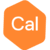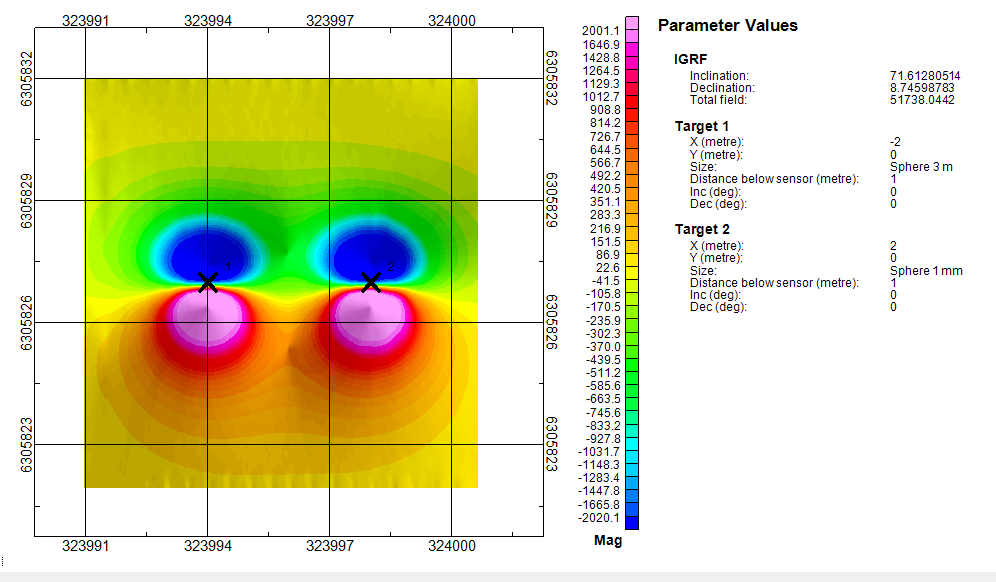# Questions about UXO Land extension forward modelling custom munitionPosts: 1Hello!

I was editing the "UxaForwardModelTable.xml" file to add new munitions for forward modelling as per UXO Land extension documentation, but noticed that some lines of the code are not doing anything. It seems that the only parameter controlling the magnetic signature is the <Magsize> tag. I added two test munitions: 1) one with the size of 3x3x3 meters (Length x Width x Tickness), 2) and another one of 1x1x1 millimeter size, for extreme comparison. The Magsize for both of these was set to 0.5. The forward modelling creates identical magnetic signatures for both munitions.So I have two questions:
1. The documentation explains that "For the magnetic response, the mag Size parameter is a scaled magnetic moment.". Is there some more documentation that explains this and how it is implemented in the code in more detail?
2. How can I make elongated munition, i.e., like a metal pipe, since the <length> tag doesn't control anything? It obviously would create a different mag signature than a round munition.

Here is the code I used.

<?xml version="1.0" encoding="utf-8"?>
<Munitions>
...
<Munition>
<Type>Sphere</Type>
<Description>Sphere 3 m</Description>
<Length unit="mm">3000</Length>
<Width unit="mm">3000</Width>
<Thickness unit="mm">3000</Thickness>
<Gates>
<Gate name="Gate 1" gatetime="0.216" unit="ms">
<Betas>
<Beta index="1">61.279</Beta>
<Beta index="2">48.67</Beta>
<Beta index="3">25.267</Beta>
</Betas>
</Gate>
<Gate name="Gate 2" gatetime="0.366" unit="ms">
<Betas>
<Beta index="1">51.967</Beta>
<Beta index="2">41.141</Beta>
<Beta index="3">18.604</Beta>
</Betas>
</Gate>
<Gate name="Gate 3" gatetime="0.660" unit="ms">
<Betas>
<Beta index="1">40.361</Beta>
<Beta index="2">31.753</Beta>
<Beta index="3">12.244</Beta>
</Betas>
</Gate>
</Gates>
<MagSize>0.5</MagSize>
</Munition>
<Munition>
<Type>Sphere</Type>
<Description>Sphere 1 mm</Description>
<Length unit="mm">1</Length>
<Width unit="mm">1</Width>
<Thickness unit="mm">1</Thickness>
<Gates>
<Gate name="Gate 1" gatetime="0.216" unit="ms">
<Betas>
<Beta index="1">61.279</Beta>
<Beta index="2">48.67</Beta>
<Beta index="3">25.267</Beta>
</Betas>
</Gate>
<Gate name="Gate 2" gatetime="0.366" unit="ms">
<Betas>
<Beta index="1">51.967</Beta>
<Beta index="2">41.141</Beta>
<Beta index="3">18.604</Beta>
</Betas>
</Gate>
<Gate name="Gate 3" gatetime="0.660" unit="ms">
<Betas>
<Beta index="1">40.361</Beta>
<Beta index="2">31.753</Beta>
<Beta index="3">12.244</Beta>
</Betas>
</Gate>
</Gates>
<MagSize>0.5</MagSize>
</Munition>
</Munitions>
Tagged: